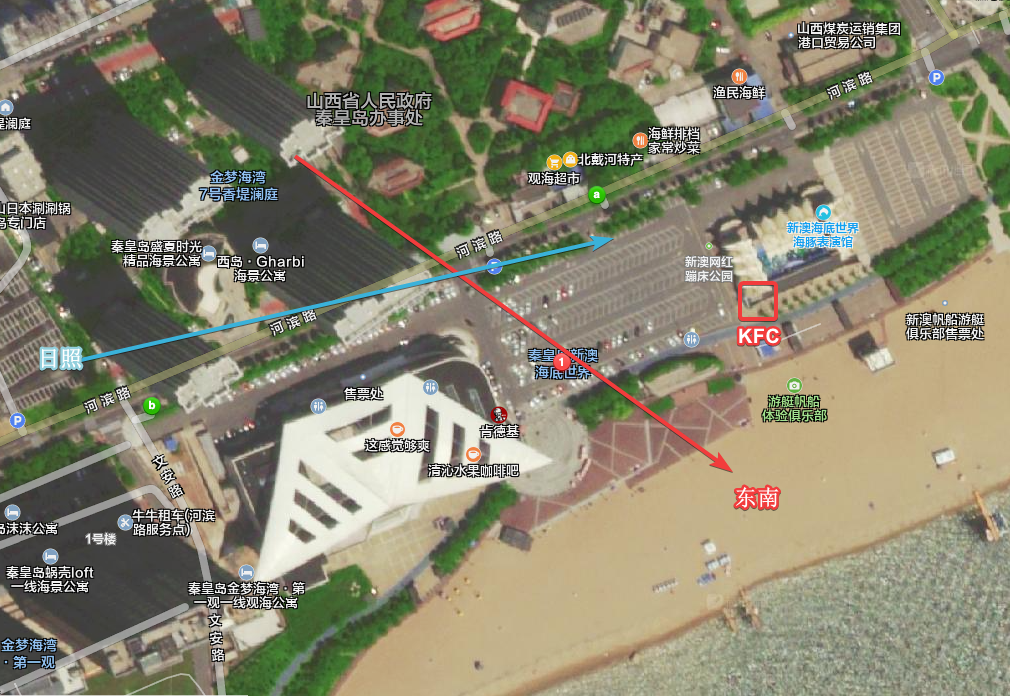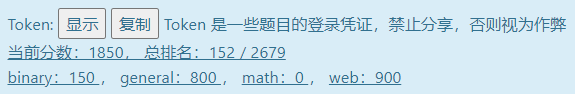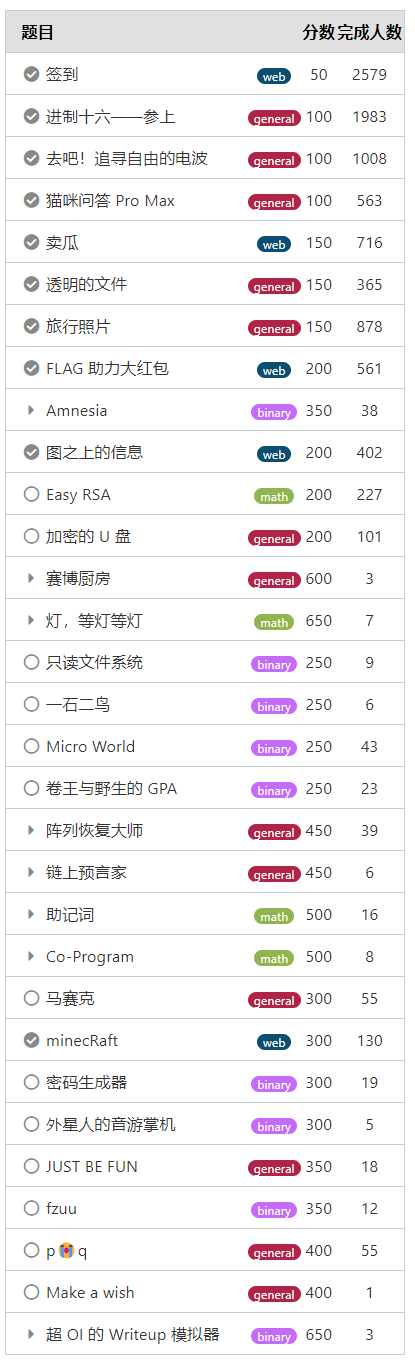## 0x1 题目

#### 签到

Hackergame 2021 比赛进行期间的任何一页

flag{HappyHacking2021-0af061d136}

#### 进制十六——参上

flag{Y0U_SH0U1D_kn0w_H0W_t0_C0nv3rt_HEX_to_TexT}

#### 去吧！追寻自由的电波

flag{phoneticab}

#### 猫咪问答 Pro Max

##### 第一题
2017 年，中科大信息安全俱乐部（[email protected]）并入中科大 Linux 用户协会（USTCLUG）。目前，信息安全俱乐部的域名（sec.ustc.edu.cn）已经无法访问，但你能找到信息安全俱乐部的社团章程在哪一天的会员代表大会上通过的吗？

##### 第二题

Google 中国科学技术大学 Linux 用户协会 五星级社团 第一条官方简介里就提到了

##### 第三题
「中国科学技术大学 Linux 用户协会位于西区图书馆的活动室门口的牌子上“LUG @ USTC”下方的小字是？」##### 第四题
「在 SIGBOVIK 2021 的一篇关于二进制 Newcomb-Benford 定律的论文中，作者一共展示了多少个数据集对其理论结果进行验证？」

Google sigbovik 2021 newcomb-benford即可找到PDF，下载后找到对应文章，作者有提到

We converted all of
the data points to their binary representations, and plotted
the relative frequency of the leading digits. The figures are
shown in Figs. 2, 3, 4, 5, 6, 7, 8, 9, 10, 11, 12, 13, and 14
in the appendix4.

##### 第五题
「不严格遵循协议规范的操作着实令人生厌，好在 IETF 于 2021 年成立了 Protocol Police 以监督并惩戒所有违背 RFC 文档的行为个体。假如你发现了某位同学可能违反了协议规范，根据 Protocol Police 相关文档中规定的举报方法，你应该将你的举报信发往何处？」

1. Reporting Offenses

wrongdoing to /dev/null. The Protocol Police are listening and will
take care of it.

flag{8804d9f3_bb1f4d1680}

#### 卖瓜

flag{HUAQIANG!HUAQIANG!_d097b45ebf}

#### 透明的文件flag{abxnniohkalmcowsayfiglet}

#### 旅行照片flag{D0n7-5hare-ph0t05-ca5ua11y}

#### FLAG 助力大红包

import requests
import time

uuid = "foobar"

for i in range(0, 256):
ip = f'{i}.0.0.0'
data = f'ip={ip}'
'X-Forwarded-For': ip,
'Content-Type': 'application/x-www-form-urlencoded',
}
print(response.text)
time.sleep(2)


flag{r3d-enve10p3-2782dacb99}

#### Amnesia

##### 轻度失忆

#include <stdio.h>
int main(void)
{
int x=0,y,*z=&y;*(z++)=0x48;*(z++)=y[x++]+0x1D;
*(z++)=y[x++]+0x07;*(z++)=y[x++]+0x00;*(z++)=y[x++]+0x03;
*(z++)=y[x++]-0x43;*(z++)=y[x++]-0x0C;*(z++)=y[x++]+0x57;
*(z++)=y[x++]-0x08;*(z++)=y[x++]+0x03;*(z++)=y[x++]-0x06;
*(z++)=y[x++]-0x08;*(z++)=y[x++]-0x43;*(z++)=y[x]-0x21;
x=*(--z); while (y[x]!=NULL) putchar (y[x++]);
return(0);
}

flag{S0_S1mp1e_r1ght_a796597e01}

#### 图之上的信息{
user(id: 1) {
privateEmail
}
}

flag{[email protected]}

#### 赛博厨房

##### Level 0

0,00,11,01,1

flag{level0_9d132008f193_5dd0c98b4e}

##### Level 1
向右 1 步

flag{level1_212d794167df_5dd0c98b4e}

#### minecRaft

    function printcinput() {
let content = document.getElementById('spann');
if (cinput === 'M') {
if (pressplateList.status === false) {
pressplateList.TurnOn_redstone_lamp();
pressplateList.status = true;
}
}
if (cinput.length >= 32) {
let tbool = gyflagh(cinput.join(''));
if (tbool) {
pressplateList.TurnOn_redstone_lamp();
content.innerText = 'Congratulations!!!';
return;
}
cinput.length = 0;
}
content.innerText = cinput.join('');
}

function gyflagh(_0x111955) {
const _0x50051f = _0x22517d; // const _0x22517d = _0x2c9e; // _0x2c9e -> _0x381b -> ['encrypt'...];
let _0x3b790d = _0x111955[_0x50051f(0x1a8)](_0x50051f(0x1b7));
if (_0x3b790d === _0x50051f(0x1aa))
return !![];
return ![];
}

function gyflagh(input) {
const _getStringFunc = getStringFunc;
let encryptResult = input.encrypt('1356853149054377');
if (encryptResult === '6fbde674819a59bfa12092565b4ca2a7a11dc670c678681daf4afb6704b82f0c') return true;
return false;
}

String['prototype']['encrypt'] = function (key) {
let i;
array_two = new Array(0x2),
array_four = new Array(0x4); // [909456177, 825439544, 892352820, 926364468]
let result = '';
let plaintext = escape(this);
for (i = 0x0; i < 0x4; i++) {
array_four[i] = Str4ToLong(key.slice(i * 0x4, (i + 0x1) * 0x4));
}
for (i = 0x0; i < plaintext.length; i += 0x8) {
array_two[0x0] = Str4ToLong(plaintext.slice(i, i + 0x4));
array_two[0x1] = Str4ToLong(plaintext.slice(i + 0x4, i + 0x8));
code(array_two, array_four);
result += LongToBase16(array_two[0x0]) + LongToBase16(array_two[0x1]);
}
return result;
}

function decrypt(key) { // key: 1356853149054377
let cipher = "6fbde674819a59bfa12092565b4ca2a7a11dc670c678681daf4afb6704b82f0c";
cipher = cipher.match(/.{1,16}/g);

const keys = new Array(0x4);
for (let i = 0x0; i < 0x4; i++) {
keys[i] = Str4ToLong(key.slice(i * 0x4, (i + 0x1) * 0x4));
}
let result = '';
for (let j = 0; j < cipher.length; j++) {
const parts = cipher[j].match(/.{1,8}/g);
parts = Base16ToLong(parts);
parts = Base16ToLong(parts);
decode(parts, keys);
parts = LongToStr4(parts)
parts = LongToStr4(parts)
result += parts + parts;
}
console.log(result);
return result;
}

code()方法：

function code(array_two, array_four) {
let part_one = array_two[0x0],
part_two = array_two[0x1];

const a = 2654435769,
b = a * 0x20;
let i = 0x0;
while (i != b) {
part_one += (part_two << 0x4 ^ part_two >>> 0x5) +
part_two ^ i +
array_four[i & 0x3];

i += a;

part_two += (part_one << 0x4 ^ part_one >>> 0x5) +
part_one ^ i +
array_four[i >>> 0xb & 0x3];
}
array_two[0x0] = part_one, array_two[0x1] = part_two;
}

function decode(array_two, array_four) {
let part_one = array_two[0x0],
part_two = array_two[0x1];

const a = 2654435769,
b = a * 0x20;

let i = b;
while (i != 0x0) {
part_two -= ((part_one << 0x4 ^ part_one >>> 0x5) +
part_one ^ i +
array_four[i >>> 0xb & 0x3]);

i -= a;

part_one -= ((part_two << 0x4 ^ part_two >>> 0x5) +
part_two ^ i +
array_four[i & 0x3]);
}
array_two[0x0] = part_one, array_two[0x1] = part_two;
}

McWebRE_inMlnCrA1t_3a5y_1cIuop9i

## 0x2 尾巴